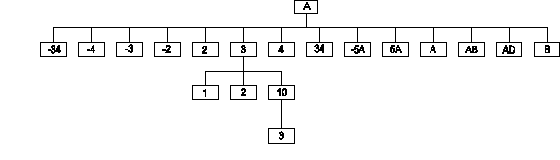# M[UMPS] Functions - \$O[RDER]

Introduced in the 1984 ANSI M[UMPS] language standard.

This function returns information about other defined information in a data-structure, based on the collating sequence in use for that data-structure.

Assume that of global variable ^A the following elements exist (see diagram):The code SET ID=-1 FOR K=1:1:25 SET ID=\$ORDER(^A(ID)) will cause the following values to be assigned to the local variable ID:
"2", "3", "4", "34", "-5A", "5A", "A", "AB", "AD", "B", "", "-34", "-4", "-3", "-2", "2", "3", "4", "34", "-5A", "5A", "A", "AB", "AD" and "B".

Reference Value \$ORDER(^A(-34)) -4 \$ORDER(^A(34)) "-5A" \$ORDER(^A("B")) "" \$ORDER(^A(3,1)) 2 \$ORDER(^A(3,10)) "" \$ORDER(^A(3,10,"")) 3 \$ORDER(^A(3,10,3)) "" \$ORDER(^A(-2)) 2 \$ORDER(^A("-5A")) "5A"

Assume that a database contains a set of customer records, organized by name of customers

^Demog("AlphaName","BAKER")=988
^Demog("AlphaName","HUSKINS")=12345
^Demog("AlphaName","ROSSMAN")=123
^Demog("AlphaName","SHIMPACH")=345
^Demog("AlphaName","SOUTHERN")=876
^Demog("AlphaName","XANDER")=1098

In order to process only a specific sub-range of that data-set, code like the following segment could be considered:

Write "Enter first letter(s): " Read start
Set name=start,done=0,index="AlphaName"
For  Do  Quit:done
. Do:\$Data(^Demog(index,name))
. . ; Process one customer
. . Quit
. Set name=\$Order(^Demog(index,name))
. Set:\$Extract(name,1,\$Length(start)'=start done=1
. Quit

Of course, it is always possible that there are multiple customers with the same name, in which case it would make sense to organize the data as:

^Demog("AlphaName","BAKER",988)=""
^Demog("AlphaName","HUSKINS",12345)=""
^Demog("AlphaName","HUSKINS",12399)=""
^Demog("AlphaName","ROSSMAN",123)=""
^Demog("AlphaName","SHIMPACH",345)=""
^Demog("AlphaName","SOUTHERN",876)=""
^Demog("AlphaName","XANDER",106)=""
^Demog("AlphaName","XANDER",1098)=""

Note that in this structure all information is in the subscripts, and that the data-value, equal to empty strings for all elements, is really irrelevant.

To process a sub-set, code like the following segment could be used:

Write "Enter first letter(s): " Read start
Set name=start,done=0,index="AlphaName"
For  Do  Quit:done
. Set cust="" For  Do  Quit:cust=""
. . Set cust=\$Order(^Demog(index,name,cust))
. . Quit:cust=""
. . ; Process one customer
. . Quit
. Set name=\$Order(^Demog(index,name))
. Set:\$Extract(name,1,\$Length(start)'=start done=1
. Quit

Addition in the 1995 ANSI M[UMPS] language standard (reverse traversal):

Reference Value \$ORDER(^A(3,1),1) 2 \$ORDER(^A(3,10),1) "" \$ORDER(^A(3,10,""),1) 3 \$ORDER(^A(3,10,3),1) "" \$ORDER(^A(3,1),-1) "" \$ORDER(^A(3,10),-1) 2 \$ORDER(^A(3,10,""),-1) 3 \$ORDER(^A(""),-1) "B"

Examples with naked references:

\$ORDER(GLVN)
SET ^ABC(1,2)="reset naked indicator"
; Naked indicator is now ^ABC(1,
SET ^(3,4)=\$ORDER(^(5,6))

; 1. fetch ^(5,6) = ^ABC(1,5,6)
; 2. store ^(3,4) = ^ABC(1,5,3,4)
; Naked indicator is now: ^ABC(1,5,3,

This document is © Ed de Moel, 1995-2005.
It is part of a book by Ed de Moel that is published under the title "M[UMPS] by Example" (ISBN 0-918118-42-5).
Printed copies of the book are no longer available.